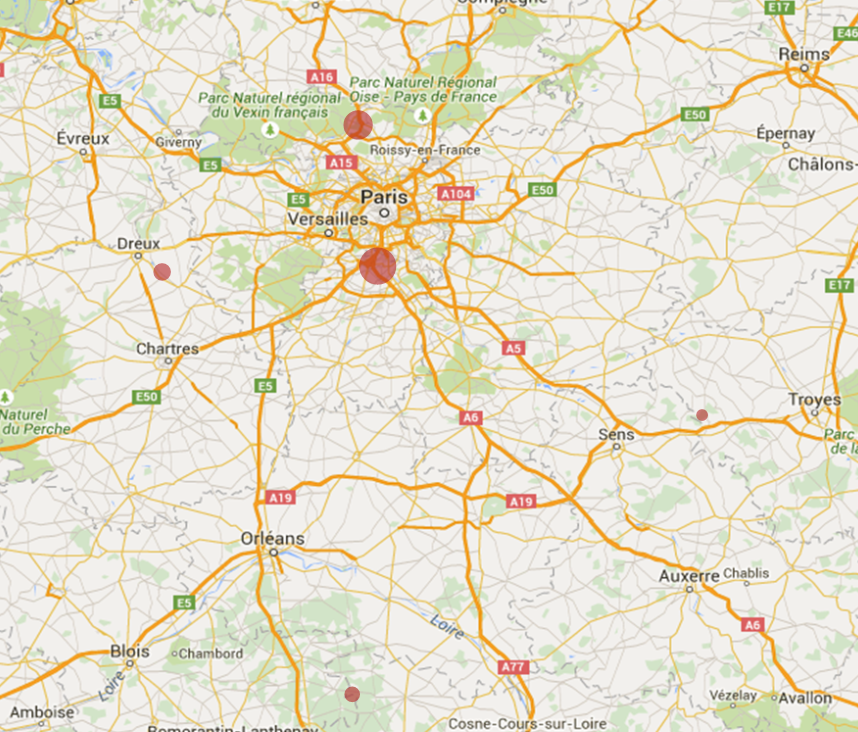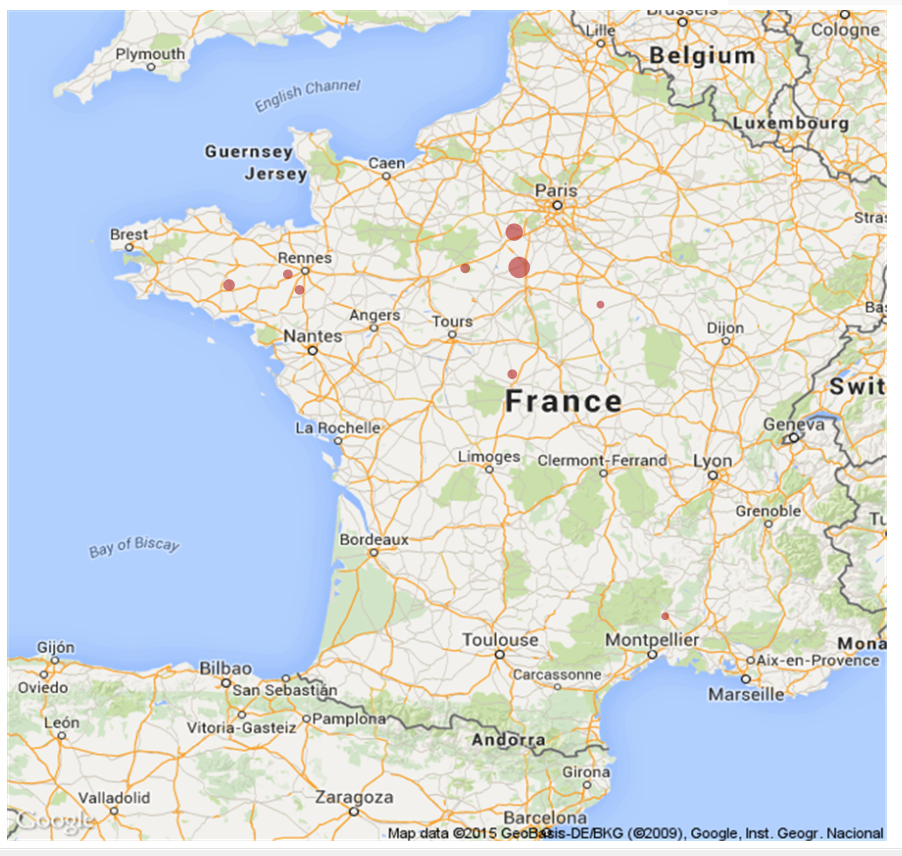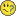# QlikView App Dev

Discussion Board for collaboration related to QlikView App Development.

Announcements
Skip the ticket, Chat with Qlik Support instead for instant assistance.
cancel
Showing results for
Did you mean:Not applicable

Hi All,

I have an offset on all the points drawed on the Google Map, but when i zoom in, the points are better placed but not in their place, anyone know why ?

Before : (wrong)Then, after zoom : (little bit better)I followed this tutorial :

Step-by-Step Google Map API v3 integration

Thank you,

Loup

1 Solution

Accepted SolutionsMVP
 map_size_x1=       '900'; map_size_y1=     '850';

Try changing these to and see if that helps:

 map_size_x1= '640';

map_size_y1=     '400';

14 RepliesNot applicable
Author

No one has had the same issue ?MVP

Do you have a legend or borders on your chart? Try clearing out everything from the chart area and see if that helps. Can you post a screenshot of the whole chart, including the borders?

Best,

SunnyNot applicable
Author

I don't have any Legend on the chart...

Whole chart :The script :

max_zoom_level = 17; //maximum value 17

var_pi180=        '=pi()/180';

var_lat_offset1=    '0';

var_mc21=        '=256*pow(2,\$(var_zoom1))';

var_mc11=        '=256*pow(2,(\$(var_zoom1)-1))';

var_mid_lat1=    '=min([Latitude Assure])+(1+var_lat_offset)*((max([Latitude Assure])-min([Latitude Assure]))/2)';

var_mid_long1=    '=min([Longitude Assure])+(max([Longitude Assure])-min([Longitude Assure]))/2';

var_zoom1=        '=max(aggr(if(max( round(256*pow(2,(_zoom_level1 -1)))+( [Longitude Assure]  *((256*pow(2,_zoom_level1 ))/360)) )-min( round(256*pow(2,(_zoom_level1 -1)))+( [Longitude Assure]  *((256*pow(2,_zoom_level1 ))/360)) ) <map_size_x1 AND max((256*pow(2,(_zoom_level1-1)))+((0.5*log((1+(sin(([Latitude Assure])*pi()/180)))/(1-(sin(([Latitude Assure])*pi()/180)))))*((-256*pow(2,_zoom_level1))/(2*pi()))))-min((256*pow(2,(_zoom_level1-1)))+((0.5*log((1+(sin(([Latitude Assure])*pi()/180)))/(1-(sin(([Latitude Assure])*pi()/180)))))*((-256*pow(2,_zoom_level1))/(2*pi()))))<map_size_y1,_zoom_level1,1),_zoom_level1))';

//var_zoom=        '5';

var_maptype1=    '=if(isnull(only(maptype)),fieldvalue( '&chr(39)&'maptype'&chr(39)&', 1 ),maptype)';

map_size_x1=       '900';

map_size_y1=     '850';

SET HidePrefix='_' ;

// Field required for calcualting best zoom level

_zoom_level1:

Load RecNo( ) as _zoom_level1 autogenerate(max_zoom_level);

@

set hideprefix = 'maptype';

Thank youMVP
 map_size_x1=       '900'; map_size_y1=     '850';

Try changing these to and see if that helps:

 map_size_x1= '640';

map_size_y1=     '400';Not applicable
Author

It is good !

So I have to change the size in order to keep this ratio ?

Thank you !

EDIT: Seems that I have to keep theses little dimensions...MVP

No problemJust in the script, I think. Front end object can stay and looks the same.

Best,

SunnyMVP
 EDIT: Seems that I have to keep theses little dimensions...

Not sure what dimensions you are talking about, can you please elaborate?Not applicable
Author

Sorry, I was speaking about the size and not dimension (=in french).

If I keep the same front end object, it look horrible (in fact the map is extented), but when I change both size (script and front end), the offset appears, so I can't have a bigger mapMVP

Would you be able to share the application? I can take a look at it, if you want.

Best,

SunnyCommunity Browser